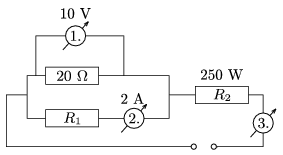Mathematical and Physical Journal
for High Schools
Issued by the MATFUND Foundation
 Already signed up? New to KöMaL?

#Problem P. 4136. (February 2009)

P. 4136. The power in the resistor R2 shown in the figure is 250 W. What are the resistances of the resistors R1 and R2? What is the reading on the third meter? What is the voltage across the power supply?(3 pont)

Deadline expired on March 11, 2009.

### Statistics:

 158 students sent a solution. 3 points: 121 students. 2 points: 21 students. 1 point: 7 students. 0 point: 4 students. Unfair, not evaluated: 5 solutionss.

Problems in Physics of KöMaL, February 2009